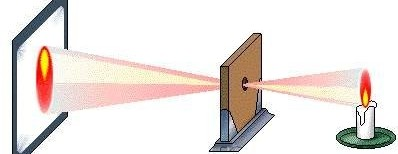# 程序设计能力实训

1055. Pinhole Imaging

When placing a board with a pinhole in the middle between an object and a screen, the reversed image of the object will appear on the screen. That is the so-called pinhole imaging.Assume that we have a candle and a screen. We know the distance $A$ between the pinhole and the candle. We also know the distance $B$ between the pinhole and the screen. We want to know the height of the fire displayed on the screen when we know the height $H$ of the real fire.

You could assume that the pinhole is very tiny and the screen is large enough.

### 输入格式

The input consists of multiple test cases. The first line of input contains an integer $T$, which is the number of test cases.

Each test case is on a separated line, and it consists three integers: $A, B, H$), separated by ONE whitespace.

$T$ is an integer, and $T \le 10$.

$A, B, H$ are integers, and $1 \le A, B, H \le 1~000~000$.

### 输出格式

For each test case, print a single line contains the height of the fire displayed on the screen, rounded to two fractional digits.

### 样例

Input
2
1 1 1
2 1 2

Output
1.00
1.00